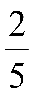A fruit buys 100kg of apples at rate of Rs.20 per kg. He sold of the apples at a loss of 7%. At what rate must the remaining apples make a profit of 15% on the sale of all apples?

# A fruit buys 100kg of apples at rate of Rs.20 per kg. He soldof the apples at a loss of 7%. At what rate must the remaining apples make a profit of 15% on the sale of all apples?

1. A
29.66
2. B
41.76
3. C
36.08
4. D
50.76

Fill Out the Form for Expert Academic Guidance!l

+91

Live ClassesBooksTest SeriesSelf Learning

Verify OTP Code (required)

### Solution:

We have cost price of apple = Rs.20 per kg
Cost price of 100kg apples = Rs.100 20
= Rs.2000apples of 100kg =kgs
Selling price of 40kg = 93 percentage of CP of 40kg
== Rs.744
Required selling price to get total profit 15 percentage = 115 percentage of total cost price
== Rs.2300
selling price of remaining 60kg = Rs.2300 744= Rs.1556
Required profit 1556 1200 = Rs.356
Profit percentage == 29.66%
Hence option (A) is correct.

## Related content

 Area of Square Area of Isosceles Triangle Pythagoras Theorem Triangle Formula Perimeter of Triangle Formula Area Formulae Volume of Cone Formula Matrices and Determinants_mathematics Critical Points Solved Examples Type of relations_mathematics+91

Live ClassesBooksTest SeriesSelf Learning

Verify OTP Code (required)